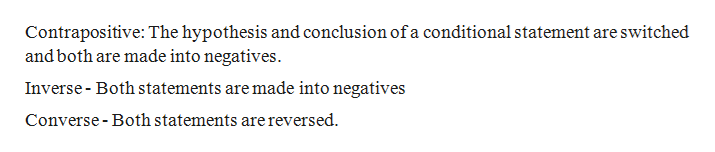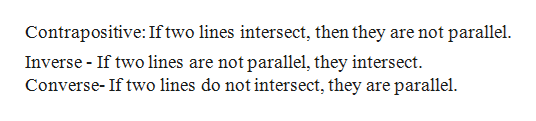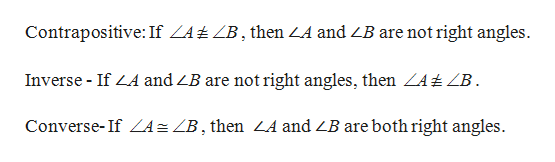# State and discuss that validity of the converse, inverse and contrapositive of each of the following statements in Euclidean geometry.If two lines are parallel, they do not interset.If ∠A and ∠B are both right angles, then ∠A ∼= ∠B.Given line m and point A not on line m, there is a circle centered at A that intersects m in at least one point.

Question
53 views
1. State and discuss that validity of the converse, inverse and contrapositive of each of the following statements in Euclidean geometry.
1. If two lines are parallel, they do not interset.
2. If ∠A and ∠B are both right angles, then ∠A = ∠B.
3. Given line m and point A not on line m, there is a circle centered at A that intersects m in at least one point.
check_circle

Step 1

Definitions:help_outlineImage TranscriptioncloseContrapositive: The hypothesis and conclusion of a conditional statement are switched and both are made into negatives Inverse- Both statements are made into negatives Converse- Both statements are reversed fullscreen
Step 2

1. If two lines are parallel, they do not intersect.help_outlineImage TranscriptioncloseContrapositive: Iftwo lines intersect, then they are not parallel Inverse If two lines are not parallel, they intersect Converse- If two lines do not intersect, they are parallel fullscreen
Step 3

2. If ∠and ∠are both right angles, then &ang...help_outlineImage TranscriptioncloseContrapositive: If ZA±ZB, then ZA and ZB are not right angles Inverse If LA and ZB are not right angles, then ZA±ZB Converse-If A= ZB, then ZA and ZB are both right angles fullscreen

### Want to see the full answer?

See Solution

#### Want to see this answer and more?

Solutions are written by subject experts who are available 24/7. Questions are typically answered within 1 hour.*

See Solution
*Response times may vary by subject and question.
Tagged in

### Geometry